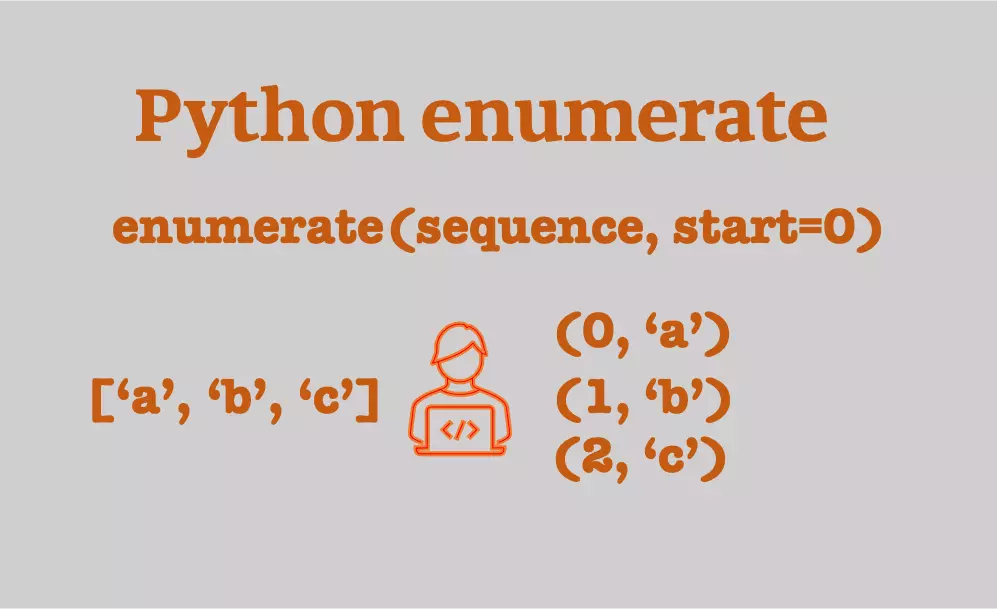# Python enumerate to simplify the looping### Python enumerate

• `enumerate` is a built-in function in Python (introduced in Python 2.3). `enumerate` allows iterating over the objects that support iteration such as a list.
• `enumerate` takes two parameters and its syntax is: `enumerate(sequence, start=0)`. It returns enumerate object containing a tuple of incremental index values and associated items.
• The advantage of the enumerate object is that the index values and items can be accessed at the same time. It helps to write cleaner and easy-to-read code (more Pythonic way).

### enumerate list

• Create the enumerate object from the list and traverse using for loop
``````# Python3 (Python 3.7.4)
x = ['python', 'R', 'julia']

for tup in enumerate(x):
print(tup)

# output
(0, 'python')
(1, 'R')
(2, 'julia')

# get index and values separately
for ind, value in enumerate(x):
print(ind, value)

# output
0 python
1 R
2 julia
``````

By default, the index values start at 0. The starting index value can be changed to 1 or to any other value.

``````x = ['python', 'R', 'julia']

# enumerate with start at 1
for tup in enumerate(x, 1):
print(tup)

# output
(1, 'python')
(2, 'R')
(3, 'julia')
``````

Get list-of-tuples or tuple-of-tuples of index values and items from the `enumerate`,

``````x = ['python', 'R', 'julia']

# get of list-of-tuples
list(enumerate(x))

# output
[(0, 'python'), (1, 'R'), (2, 'julia')]

# get of tuple-of-tuples
tuple(enumerate(x))
# output
((0, 'python'), (1, 'R'), (2, 'julia'))
``````

### Enumerate can traverse only once

• The list to `enumerate` object generates iterator which can be traversed only once
``````x = ['python', 'R', 'julia']
enum = enumerate(x)
list(enum)
# output
[(0, 'python'), (1, 'R'), (2, 'julia')]

# try again
list(enum)
# output
[]
``````

To iterate it multiple times, wrap the `enumerate` to container object such as a list or use in for loop (see above examples)

### Enumerate dictionary

• enumerate the dictionary for both keys and values
``````x = {1:'python', 2:'R', 3:'julia'}

for ind, (key, value) in enumerate(x.items()):
print(ind, key, value)

# output
0 1 python
1 2 R
2 3 julia
``````

### Enumerate strings

• Iterate each character in a string using enumerate
``````for ind, value in enumerate('python'):
print(ind, value)

# output
0 p
1 y
2 t
3 h
4 o
5 n
``````

### Enumerate example

• `enumerate` is useful to iterate over multiple lists when the index is useful
• For example, if you want to find the index of lists when items from two list matches
``````x = ['python', 'R', 'julia']
y = ['java', 'go', 'python']

for ind1, item1 in enumerate(x):
for ind2, item2 in enumerate(y):
if item1 == item2:
print(ind1, ind2)

# output
0 2
``````